Next: Solution of lunar equations Up: Lunar motion Previous: Derivation of lunar equations

# Useful definitions

At this stage, it is convenient to introduce the concept of the mean moon. This is an imaginary body that executes a circular orbit about the Earth, in the ecliptic plane, whose radius is equal to the (unperturbed) mean radius of the Moon's orbit,, and which moves at a steady angular velocity equal to the Moon's mean orbital angular velocity,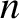. Likewise, the mean sun is a second imaginary body that executes a circular orbit about the Earth-Moon barycenter, in the ecliptic plane, whose radius is equal to the mean radius of the Sun's (apparent) orbit,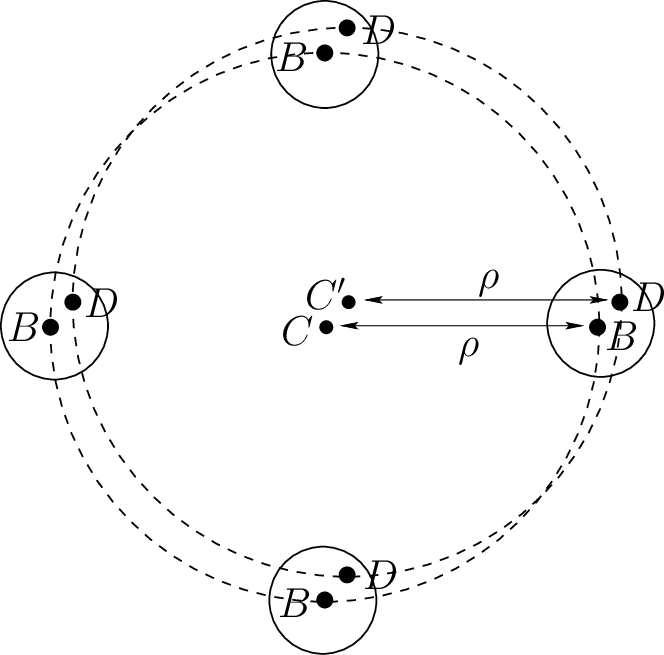, and which moves at a steady angular velocity equal to the Sun's mean orbital angular velocity,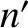. Thus, the geocentric'' ecliptic longitudes of the mean moon and the mean sun are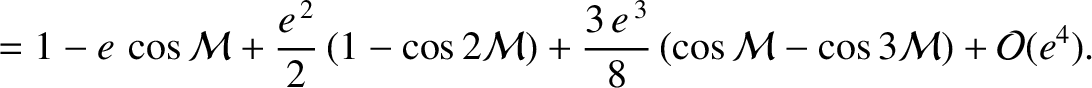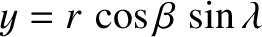(11.97) and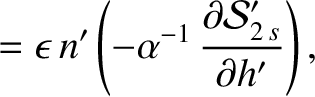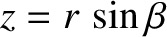(11.98)

respectively. (Actually, our geocentric'' ecliptic longitude of the Sun is measured with respect to the Earth-Moon barycenter.) Here, for the sake of simplicity, and also for the sake of consistency with our previous analysis, we have assumed that both objects are located at ecliptic longitude zero at time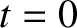.

The following definitions are useful. The lunar mean anomaly,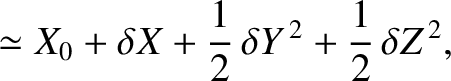(11.99)

is defined as the angular distance (in geocentric ecliptic longitude) between the mean moon and the lunar perigee. Here,is the ecliptic longitude of the lunar perigee. The lunar mean argument of latitude,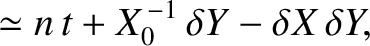(11.100)

is defined as the angular distance (in geocentric ecliptic longitude) between the mean moon and the lunar ascending node. Here,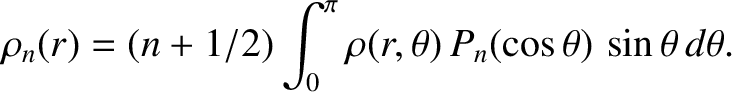is the ecliptic longitude of the lunar ascending node. The lunar mean elongation from the Sun,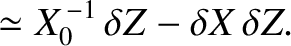(11.101)

is defined as the difference between the geocentric ecliptic longitudes of the mean moon and the mean sun. Finally, the solar mean anomaly,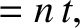(11.102)

is defined as the angular distance (in geocentric ecliptic longitude) between the mean Sun and the solar perigee. Here, for the sake of simplicity, and also for the sake of consistency with our previous analysis, we have assumed that the solar perigee lies at ecliptic longitude zero. Note that we are neglecting the precession of the solar perigee (which is really the precession of the Earth-Moon barycenter's perihelion), because such precession takes place on a far longer timescale than any characteristic timescale of the lunar motion. (See Sections 5.4 and 10.3.)

Finally, it is convenient to define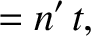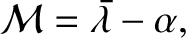(11.103)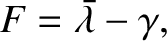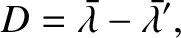(11.104) and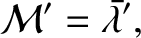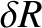(11.105)

Thus,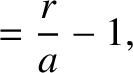is the difference between the radial distance of the Moon from the Earth and that of the mean moon from the Earth,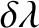is the difference between the geocentric ecliptic longitudes of the Moon and the mean moon, and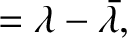is the difference between the geocentric ecliptic latitudes of the Moon and the mean moon.Next: Solution of lunar equations Up: Lunar motion Previous: Derivation of lunar equations
Richard Fitzpatrick 2016-03-31